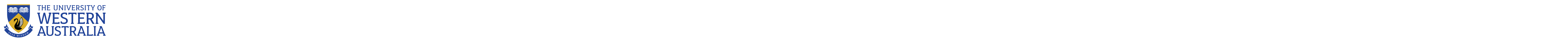School of Mathematics and Statistics

# School of Mathematics and Statistics

Research being undertaken by our current postgraduates:

Michael Pauley

### Michael Pauley

#### Thesis

Riemannian cubics

#### Summary

Suppose we are given a sequence of points and want to know a smooth curve which interpolates these points. In Euclidean space, a cubic spline is an appropriate interpolant. In many problems, however, the space of interest is not Euclidean space, but some Riemannian manifold with curvature, such as the group of rotations. Cubics can be generalised to such spaces by choosing the extremal curves of the mean square covariant acceleration. The resulting curves are called Riemannian cubics. I am studying the differential equations related to Riemannian cubics in Lie groups.

#### Why my research is important

It is important to be able to deal with processes for which the laws describing them are only known approximately. Riemannian cubics can be a useful tool for such situations. Riemannian cubics are also useful in computer graphics, and in trajectory planning for rigid body motion: for example the motion of a mechanical arm may be described by a curve in the group of rotations, and Riemannian cubics can be used to control the arm in as smooth a way as possible.

### Statistics clinic

Assistance in statistics is available for Postgraduates students by research at the UWA Centre for Applied Statistics.

# The University of Western Australia

## University information

CRICOS Code: 00126G